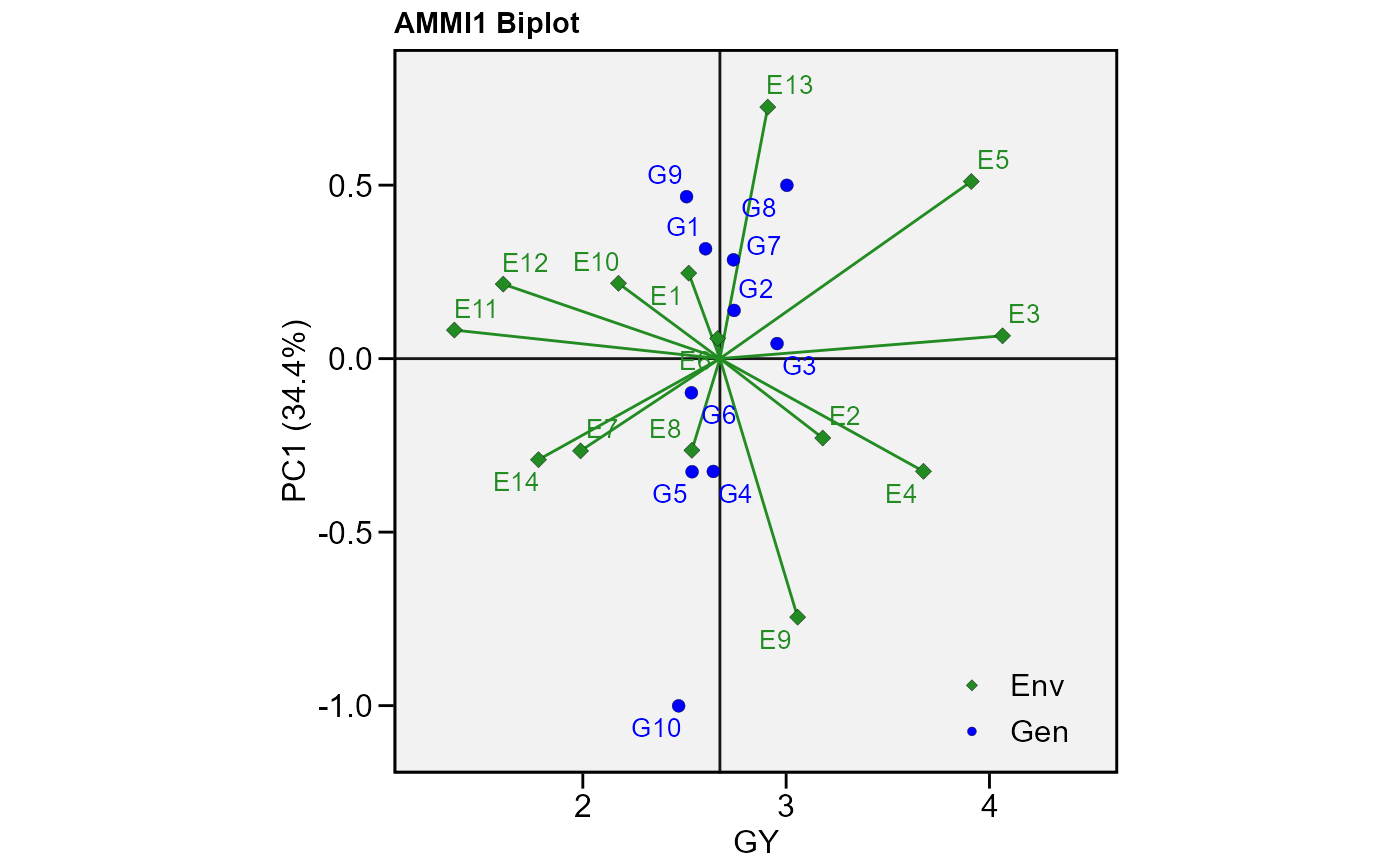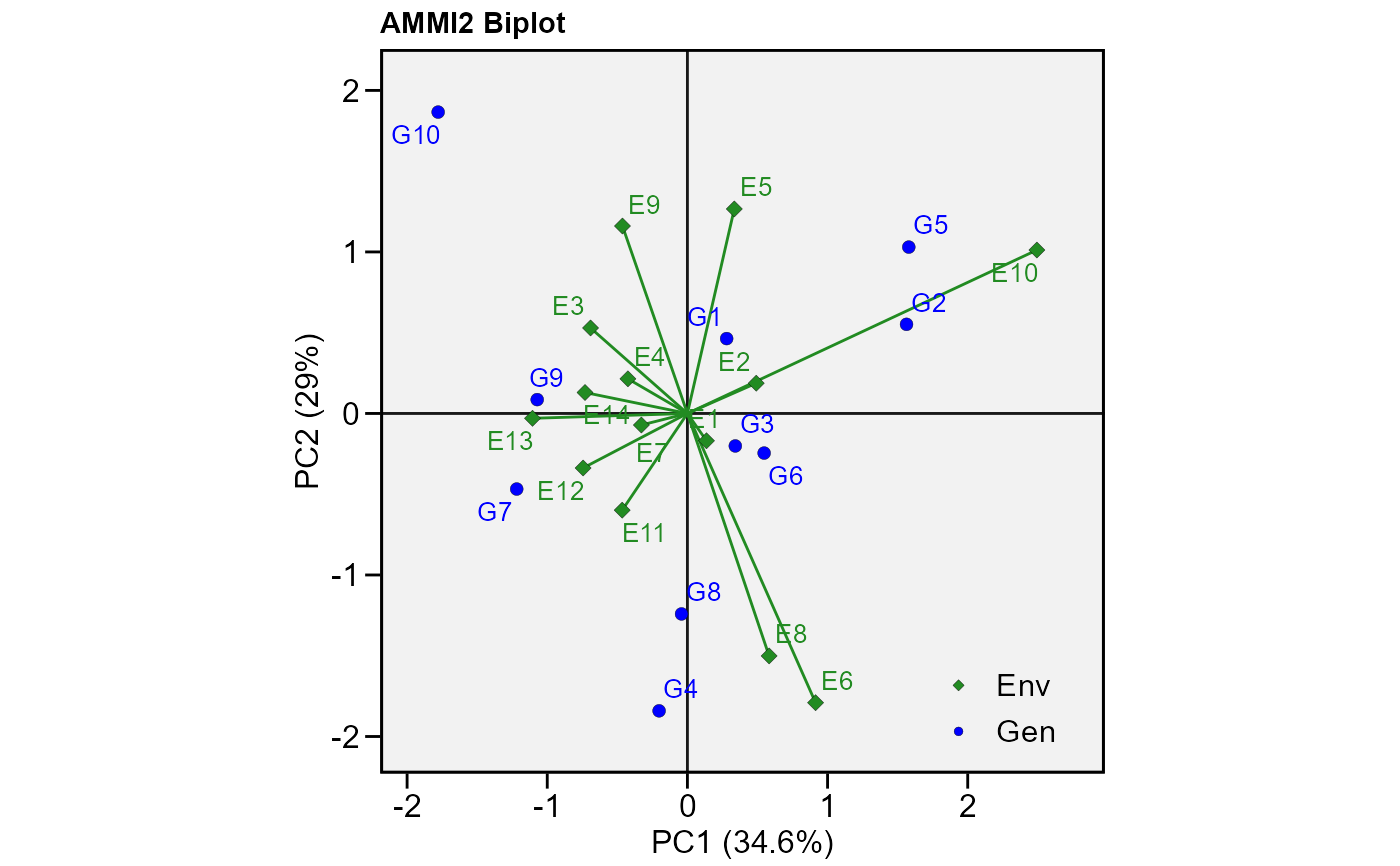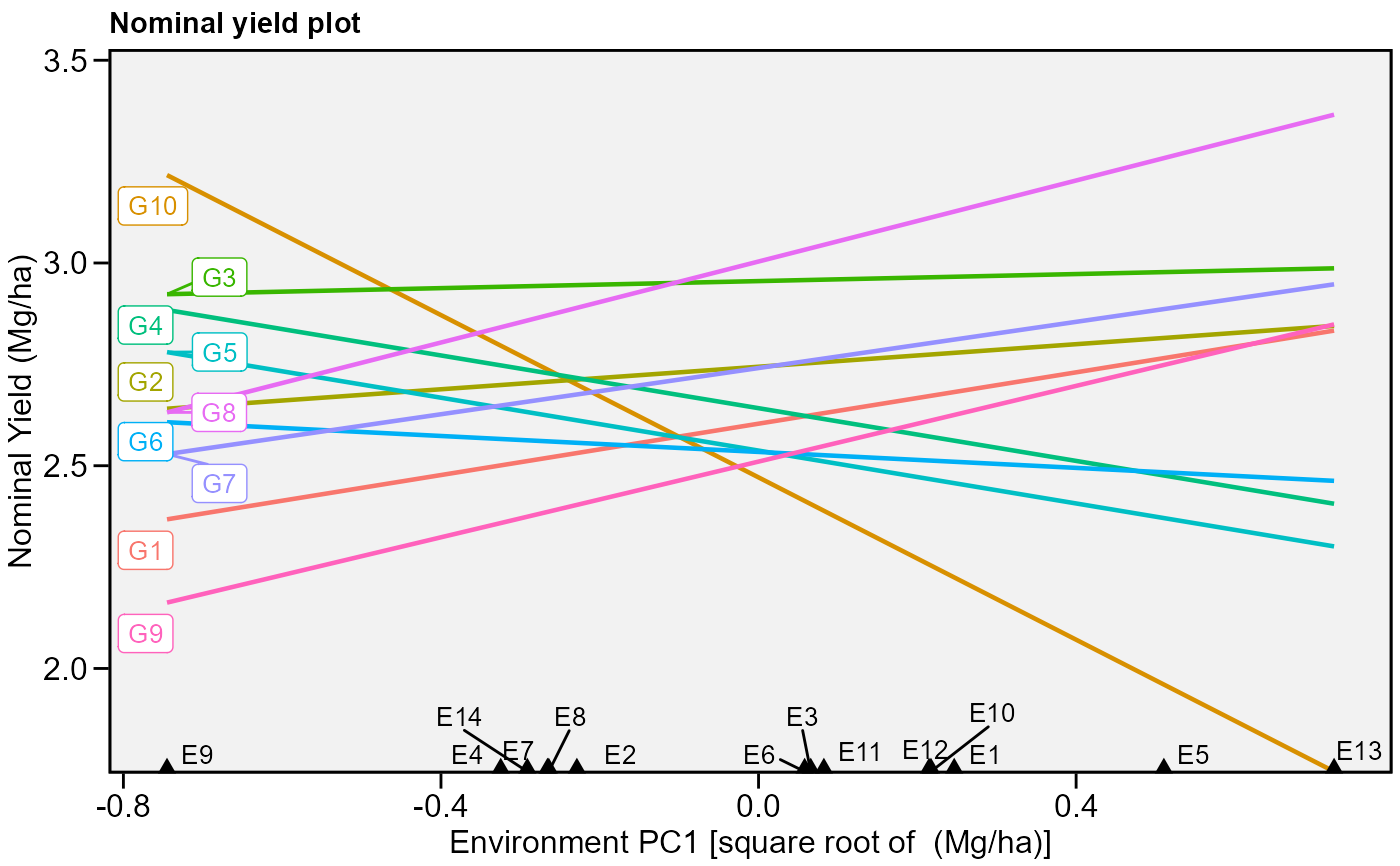Compute the Additive Main effects and Multiplicative interaction. This function also serves as a helper function for other procedures performed in the metan package such as waas and wsmp

performs_ammi(.data, env, gen, rep, resp, verbose = TRUE)

## Arguments

.data The dataset containing the columns related to Environments, Genotypes, replication/block and response variable(s). The name of the column that contains the levels of the environments The name of the column that contains the levels of the genotypes The name of the column that contains the levels of the replications/blocks The response variable(s). To analyze multiple variables in a single procedure a vector of variables may be used. For example resp = c(var1, var2, var3). Logical argument. If verbose = FALSE the code will run silently.

## Value

• ANOVA The analysis of variance for the AMMI model.

• PCA The principal component analysis

• MeansGxE The means of genotypes in the environments

• model scores for genotypes and environments in all the possible axes.

## Examples


library(metan)
ammi_model = performs_ammi(data_ge, ENV, GEN, REP,
resp = c(GY, HM))#> Evaluating variable GY 50 %
#> Evaluating variable HM 100 %
#> All variables with significant (p < 0.05) genotype-vs-environment interaction
#> Done!
# GY x PC1 (variable GY)
plot_scores(ammi_model$GY, col.env = 'olivedrab', col.gen = 'orange2', x.lab = 'My own x label')# PC1 x PC2 (variable HM) plot_scores(ammi_model$HM,
type = 2)# PC1 x PC2 (variable HM)
# Draw a convex hull polygon
plot_scores(ammi_model\$HM,
type = 2,
polygon = TRUE)